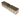06.10.2020 — Category 1

# Trying out prismic

```# Belt-concrete pattern for factorio
/silent-command

game.player.print("Producing Belt Pattern")
local pos = {
x = game.player.position.x + 2,
y = game.player.position.y
}
local newTiles = {}

local boundingBox = {
y1=pos.y - 0,
y2=pos.y + 1,
x1=pos.x - 1,
x2=pos.x + 400
}

for y=boundingBox.y1,boundingBox.y2 do
for x=boundingBox.x1,boundingBox.x2 do
table.insert(newTiles, {name="refined-hazard-concrete-left", position={x, y}})
end
end

game.players.surface.set_tiles(newTiles)```

A quote block, whats this look like?

- Wandering dev